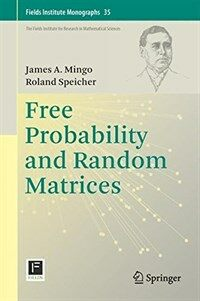> 상세정보

상세정보Free probability and random matrices [electronic resource]

자료유형
E-Book(소장)
개인저자
Mingo, James A. Speicher, Roland.
서명 / 저자사항
Free probability and random matrices [electronic resource] / James A. Mingo, Roland Speicher.
발행사항
New York :   Springer,   c2017.
형태사항
1 online resource (xiv, 336 p.) : ill.
총서사항
Fields Institute Monographs,1069-5273 ; 35
ISBN
9781493969418 9781493969425 (e-book)
요약
This volume opens the world of free probability to a wide variety of readers. From its roots in the theory of operator algebras, free probability has intertwined with non-crossing partitions, random matrices, applications in wireless communications, representation theory of large groups, quantum groups, the invariant subspace problem, large deviations, subfactors, and beyond. This book puts a special emphasis on the relation of free probability to random matrices, but also touches upon the operator algebraic, combinatorial, and analytic aspects of the theory. The book serves as a combination textbook/research monograph, with self-contained chapters, exercises scattered throughout the text, and coverage of important ongoing progress of the theory. It will appeal to graduate students and all mathematicians interested in random matrices and free probability from the point of view of operator algebras, combinatorics, analytic functions, or applications in engineering and statistical physics.
일반주기
Title from e-Book title page.
내용주기
1. Asymptotic Freeness of Gaussian Random Matrices -- 2. The Free Central Limit Theorem and Free Cumulants -- 3. Free Harmonic Analysis -- 4. Asymptotic Freeness -- 5. Second Order Freeness -- 6. Free Group Factors and Freeness -- 7. Free Entropy X-the Microstates Approach via Large Deviations -- Free Entropy X*-the Non-Microstates Approach via Free Fisher Information -- 9. Operator-Valued Free Probability Theory and Block Random Matrices -- 10. Polynomials in Free Variables and Operator-Valued Convolution -- 11. Brown Measure -- Solutions to Exercises -- References -- Index of Exercises.
서지주기
Includes bibliographical references and index.
이용가능한 다른형태자료
Issued also as a book.
일반주제명
Free probability theory. Random matrices. Functional analysis.
바로가기
 000 00000cam u2200205 a 4500 001 000045993552 005 20190813152659 006 m d 007 cr 008 190806s2017 nyua ob 001 0 eng d 020 ▼a 9781493969418 020 ▼a 9781493969425 (e-book) 040 ▼a 211009 ▼c 211009 ▼d 211009 050 0 0 ▼a QA326 082 0 4 ▼a 512.55 ▼2 22 084 ▼a 512.55 ▼2 DDCK 090 ▼a 512.55 100 1 ▼a Mingo, James A. 245 1 0 ▼a Free probability and random matrices ▼h [electronic resource] / ▼c James A. Mingo, Roland Speicher. 260 ▼a New York : ▼b Springer, ▼c c2017. 300 ▼a 1 online resource (xiv, 336 p.) : ▼b ill. 490 1 ▼a Fields Institute Monographs, ▼x 1069-5273 ; ▼v 35 500 ▼a Title from e-Book title page. 504 ▼a Includes bibliographical references and index. 505 0 ▼a 1. Asymptotic Freeness of Gaussian Random Matrices -- 2. The Free Central Limit Theorem and Free Cumulants -- 3. Free Harmonic Analysis -- 4. Asymptotic Freeness -- 5. Second Order Freeness -- 6. Free Group Factors and Freeness -- 7. Free Entropy X-the Microstates Approach via Large Deviations -- Free Entropy X*-the Non-Microstates Approach via Free Fisher Information -- 9. Operator-Valued Free Probability Theory and Block Random Matrices -- 10. Polynomials in Free Variables and Operator-Valued Convolution -- 11. Brown Measure -- Solutions to Exercises -- References -- Index of Exercises. 520 ▼a This volume opens the world of free probability to a wide variety of readers. From its roots in the theory of operator algebras, free probability has intertwined with non-crossing partitions, random matrices, applications in wireless communications, representation theory of large groups, quantum groups, the invariant subspace problem, large deviations, subfactors, and beyond. This book puts a special emphasis on the relation of free probability to random matrices, but also touches upon the operator algebraic, combinatorial, and analytic aspects of the theory. The book serves as a combination textbook/research monograph, with self-contained chapters, exercises scattered throughout the text, and coverage of important ongoing progress of the theory. It will appeal to graduate students and all mathematicians interested in random matrices and free probability from the point of view of operator algebras, combinatorics, analytic functions, or applications in engineering and statistical physics. 530 ▼a Issued also as a book. 538 ▼a Mode of access: World Wide Web. 650 0 ▼a Free probability theory. 650 0 ▼a Random matrices. 650 0 ▼a Functional analysis. 700 1 ▼a Speicher, Roland. 830 0 ▼a Fields Institute Monographs; ▼v 35. 856 4 0 ▼u https://oca.korea.ac.kr/link.n2s?url=https://doi.org/10.1007/978-1-4939-6942-5 945 ▼a KLPA 991 ▼a E-Book(소장)

소장정보

No. 소장처 청구기호 등록번호 도서상태 반납예정일 예약 서비스
No. 1 소장처 청구기호 CR 512.55 등록번호 E14016758 도서상태 대출불가(열람가능) 반납예정일 예약 서비스

컨텐츠정보

목차

1. Asymptotic Freeness of Gaussian Random Matrices.- 2. The Free Central Limit Theorem and Free Cumulants.- 3. Free Harmonic Analysis.- 4. Asymptotic Freeness.- 5. Second Order Freeness.- 6. Free Group Factors and Freeness.- 7. Free Entropy X-the Microstates Approach via Large Deviations.- Free Entropy X -the Non-Microstates Approach via Free Fisher Information.- 9. Operator-Valued Free Probability Theory and Block Random Matrices.- 10. Polynomials in Free Variables and Operator-Valued Convolution.- 11. Brown Measure.- Solutions to Exercises.- References.- Index of Exercises.

관련분야 신착자료

Linear and geometric algebra

Macdonald, Alan (2021)